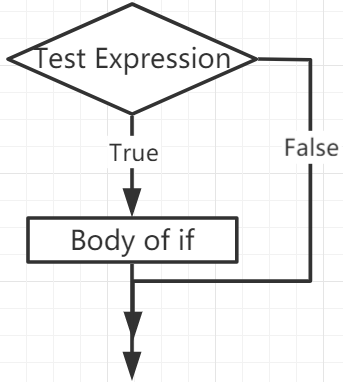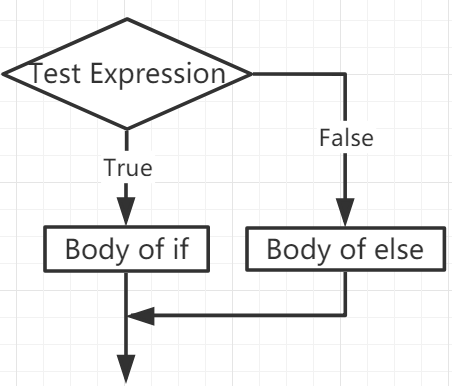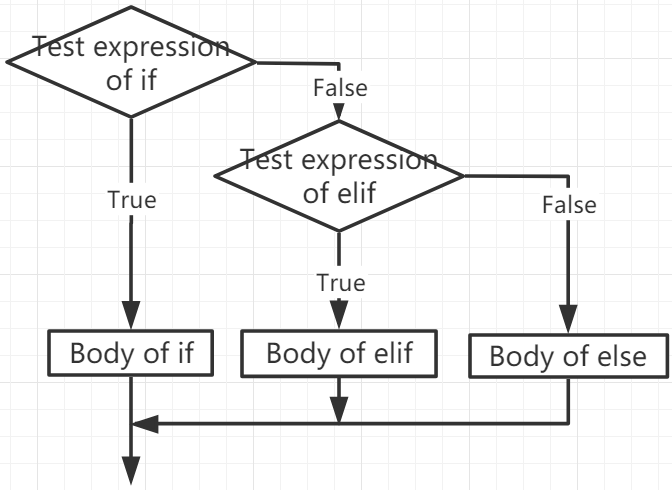# If Else¶

Decision making is required when we want to execute a code only if a certain condition is satisfied.

## if¶

```if test expression:
statement(s)
```

Here, the program evaluates the `test expression` and executes the `statement` only when the `test expression` is True.

If `test expression` is False, then `statement(s)` will not be executed.

In MicroPython, indentation means the body of the `if` statement. The body starts with an indentation and ends with the first unindented line.

Python interprets non-zero values ​​as “True”. None and 0 are interpreted as “False”.

if Statement FlowchartExample

```num = 8
if num > 0:
print(num, "is a positive number.")
print("End with this line")
```
```>>> %Run -c \$EDITOR_CONTENT
8 is a positive number.
End with this line
```

## if…else¶

```if test expression:
Body of if
else:
Body of else
```

The `if..else` statement evaluates `test expression` and will execute the body of `if` only when the test condition is `True`.

If the condition is `False`, the body of `else` is executed. Indentation is used to separate the blocks.

if…else Statement FlowchartExample

```num = -8
if num > 0:
print(num, "is a positive number.")
else:
print(num, "is a negative number.")
```
```>>> %Run -c \$EDITOR_CONTENT
-8 is a negative number.
```

## if…elif…else¶

```if test expression:
Body of if
elif test expression:
Body of elif
else:
Body of else
```

`Elif` is short for `else if`. It allows us to check multiple expressions.

If the condition of the `if` is False, the condition of the next elif block is checked, and so on.

If all conditions are `False`, the body of `else` is executed.

Only one of several `if...elif...else` blocks is executed according to the conditions.

The `if` block can only have one `else` block. But it can have multiple `elif` blocks.

if…elif…else Statement FlowchartExample

```x = 10
y = 9

if x > y:
print("x is greater than y")
elif x == y:
print("x and y are equal")
else:
print("x is greater than y")
```
```>>> %Run -c \$EDITOR_CONTENT
x is greater than y
```

## Nested if¶

We can embed an if statement into another if statement, and then call it a nested if statement.

Example

```x = 67

if x > 10:
print("Above ten,")
if x > 20:
print("and also above 20!")
else:
print("but not above 20.")
```
```>>> %Run -c \$EDITOR_CONTENT
Above ten,
and also above 20!
```